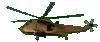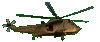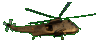Factor Trinomials      x2+bx+c Type      Fun Game Help Tips:

- When you factor the trinomial   x2+5x+6   the answer is   (x+2)(x+3) .
From the answer, notice that   '2 add 3' = 5   and that   '2 times 3' = 6 .

- In general, when you factor the trinomial   x2+bx+c   the answer is   (x+s)(x+t) ,
where the   's add t' = b   and the   's times t' = c .

- This means the question x2+bx+c can be thought of as x2+(s+t)x+st .
You can check the answer by multiplying (x+s)(x+t) together.

- Similarly, when you factor the trinomial x2-5x+6 the answer is (x-2)(x-3).
From the answer, notice that '-2 add -3' = -5 and that '-2 times -3' = +6 .

- Also, when you factor the trinomial x2-x-6 the answer is (x+2)(x-3).
From the answer, notice that '+2 add -3' = -1 and that '+2 times -3' = -6 .

- Your Game Score is reduced by the number of helicopter hits.

- To slow the game speed repeat tap/click on the word Slider.
- To increase the game speed repeat tap/click on the word Math.
- Speed can also be adjusted with a keyboard's - and + keys.

- Refresh/Reload the web page to restart the game.